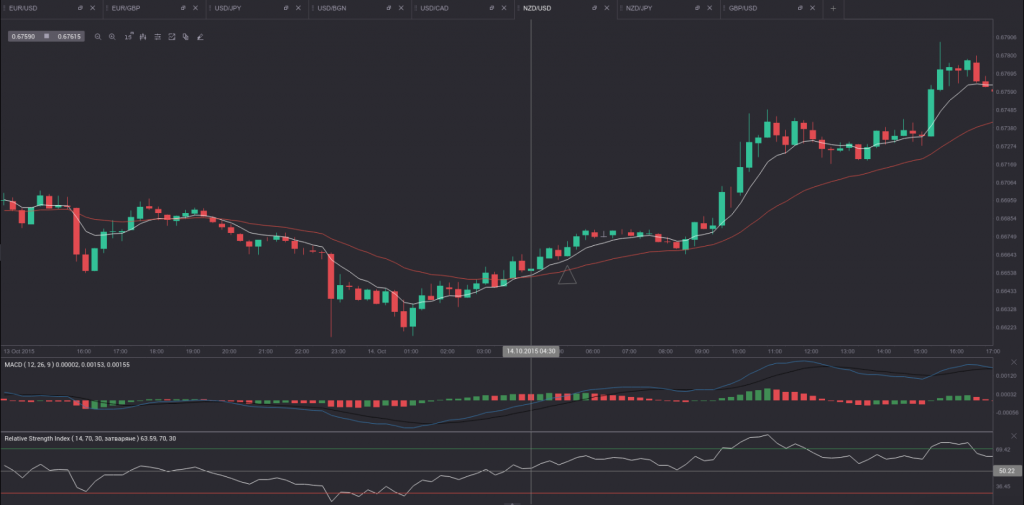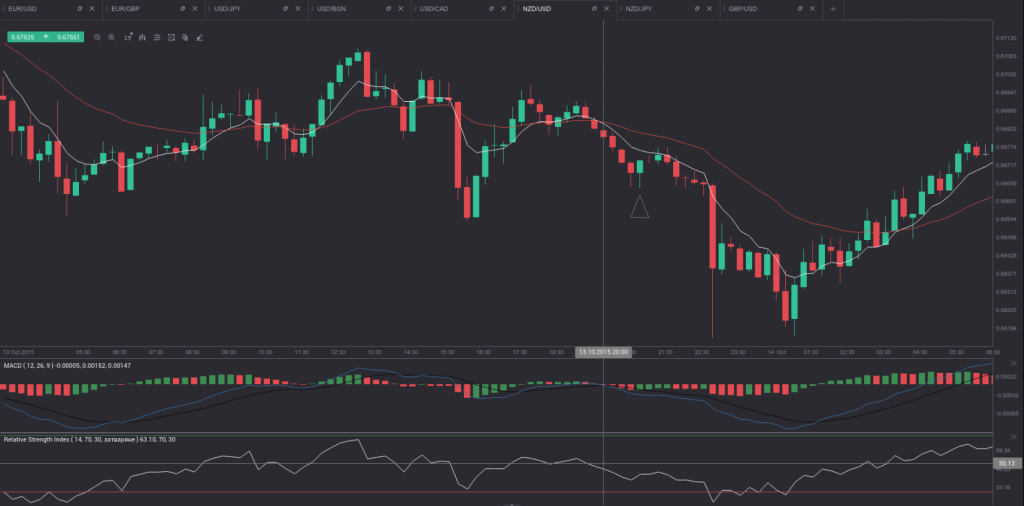# 60-minute Binary Options Strategy using EMAs, MACD and RSI

For this strategy we will utilize a combination of technical indicators – two exponential moving averages with different periods, the Moving Average Convergence Divergence (MACD) and the Relative Strength Index (RSI). We need a shorter-term Exponential Moving Average (7-day EMA, white on the charts below) and a longer-term one (26-day EMA, red on the charts below). In addition, we will use the default settings of the RSI (14 periods, 30.00 as oversold, 70.00 as overbought) and the default settings of the MACD (short-term is 12, long-term is 26 and MACD periods are 9). The time frame is set to 15 minutes, while the expiry time is 60 minutes (a four-candle expiration time).

In order to make trading decisions, one needs to take into account the following conditions:

If he/she is to buy a Call option:

1. The 7-day EMA needs to cross the 26-day EMA in a bottom-up manner,
2. The RSI needs to move above its 50.00 level and
3. The MACD line (blue on the chart below) needs to be ascending.If he/she is to buy a Put option:

1. The 7-day EMA needs to cross the 26-day EMA in a top-down manner,
2. The RSI needs to move below its 50.00 level and
3. The MACD line (blue on the chart below) needs to be descending.You should make sure that all three conditions are present before you make your entry.

On the 15-minute charts above the vertical lines mark where a call and a put entry should be made, while the triangles mark the expiry.